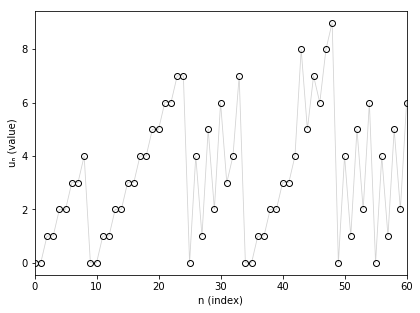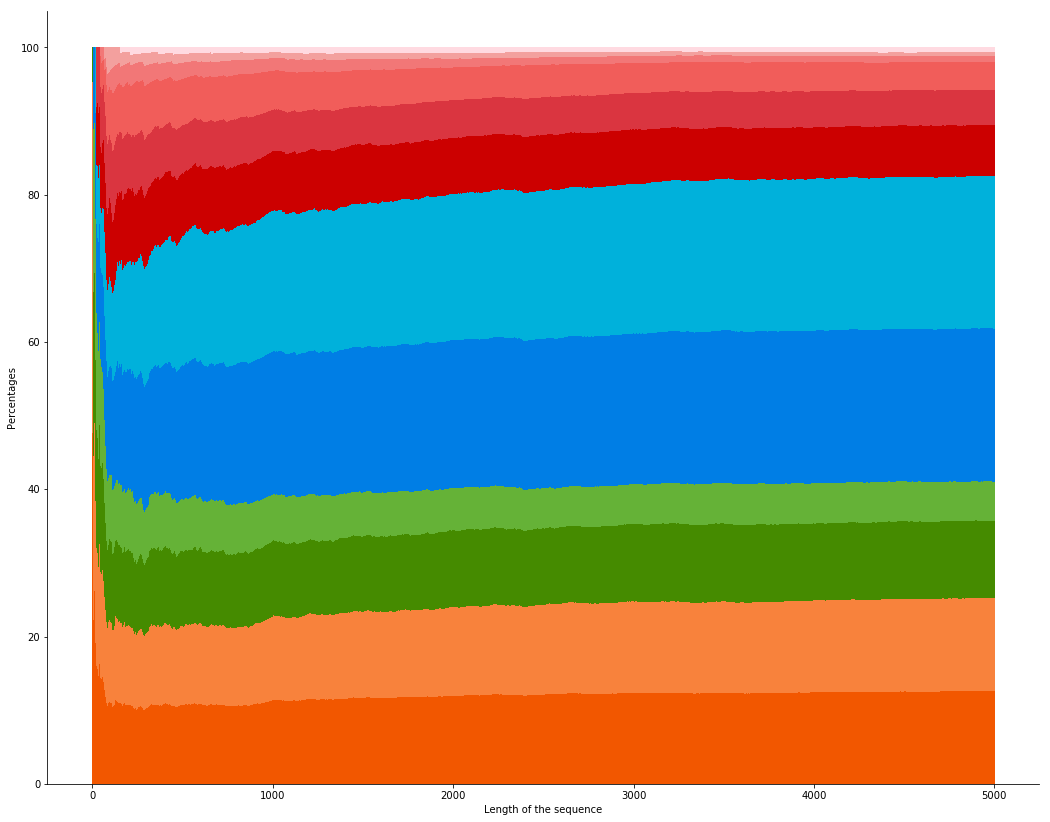# Nim function for take-a-prime game

The Nim function for take-a-prime game is a math sequence with interesting patterns. We define this sequence $$(u_n)$$ recursively. For all $$n$$ in $$\mathbb{N}$$ (the set of non-negative integers), $$u_n$$ is the lowest number in $$\mathbb{N}$$ such that for all prime numbers $$p$$ (with $$n-p \geq 0$$), $$u_n \neq u_{n-p}$$.To understand this definition, we detail how to compute the first terms. For $$n=0$$, no prime number verifies $$n-p \geq 0$$, then $$u_n$$ is the lowest number in $$\mathbb{N}$$ and $$u_0=0$$. We get the same for $$n=1$$ and obtain $$u_1=0$$.

For $$n=2$$, the only prime number $$p$$ which verifies $$n-p \geq 0$$ is $$p=2$$. Then, the condition means that we have to find the lowest number in $$\mathbb{N}$$ which is different from $$u_{n-p} = u_{2-2} = u_0 = 0$$. We finally have $$u_2=1$$.

For $$n=3$$, $$u_3$$ has to differ from $$u_{3-2}=0$$ and $$u_{3-3}=0$$, then $$u_3=1$$.

For $$n=4$$, $$u_4$$ has to differ from $$u_{4-2}=1$$ and $$u_{4-3}=0$$, then $$u_4=2$$.

For now, the sequence doesn’t seem very strange. But if we look at the first 100 terms from $$u_0$$ to $$u_{99}$$, we get (I skipped lines to reveal some patterns of the sequence):

0, 0, 1, 1, 2, 2, 3, 3, 4,
0, 0, 1, 1, 2, 2, 3, 3, 4, 4, 5, 5, 6, 6, 7, 7,
0, 4, 1, 5, 2, 6, 3, 4, 7,
0, 0, 1, 1, 2, 2, 3, 3,
4, 8, 5, 7, 6, 8, 9,
0, 4, 1, 5, 2, 6,
0, 4, 1, 5, 2, 6, 3,
4, 7, 5, 8, 4, 10, 5, 7, 6, 8,
4, 7, 5, 8, 6, 10, 9, 7,
4, 8, 5, 10, 6,
0, 4, 1, 5, 2, 6,
0, 4, 1, 5, 2, 6, 3, 4, 7.


We show that the recursion definition of the sequence makes it to adopt some patterns like “0, 4, 1, 5, 2, 6”.

To go forward in the computational way, we write a program to obtain a few million terms of the sequence (see outputs and code in C++ in my github). We can make two interesting conjectures:

• First, the proportions of 0, 1, 2, etc. in the sequence (between 0 and an integer N) seem to tend towards constant proportions (as N goes to infinity), as we can see in the following plot.Legend: stacked plot of proportions to obtain values from 0 to 11 as the length of the sequence increases. The proportions are stacked from 0 to 11: 0 is on the bottom (in dark orange) and 11 is on the top (in pink).

• Next, the sequence seems to only take values between 0 and 11. Actually, by showing at the index of the first occurence of values, we speculate that no new value (above 11) appears after the site 156.
 value site of first apparition 0 0 1 2 2 4 3 6 4 8 5 19 6 21 7 23 8 43 9 48 10 67 11 156

From a mathematical perspective, the problem seems really difficult to solve! I went to the encyclopedia of integer sequences (OEIS) and found the sequence and its name: Nim function for take-a-prime game.

A common method to go further is to generalize the problem, that is to study the sequence (a Nim function) for each subset of $$\mathbb{N}$$. Some of them are easy to solve (take $$\mathbb{N}$$ as a subset…) and others seems very chaotic (with the set of prime numbers for example). Insights have been done in the dissertation of Achim Flammenkamp (see here, “Long periods in subtraction games”), where many subsets of $$\mathbb{N}$$ are considered. But I was not able to find more information related to “my” conjectures.

Finally, we can see that if we append the number 1 in addition with the set of the prime numbers, we get a really simple pattern of “0, 1, 2, 3” (and it’s easy to prove it). On the contrary, if we remove 2 of the set of the prime numbers, we still get a sequence with strange patterns (beginning with “0, 0, 0, 1, 1, 1, 2, 2, 2, 3, 0, 3, 4, 1, 4, 3”)! Related contents:

Written on November 11, 2014